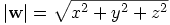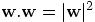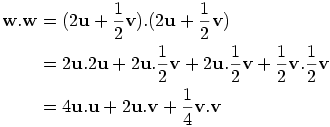## vectors

### xClairex

Posted 10 March 2007 - 09:53 PM

stuck on a few questions any help will be much appreciated

1.The triangle PQR has vertices P(1,4,-1) Q(3,4,2) and R (-2,4,1). Show that PQR is:
(i)isosceles
(ii)right-angled at P

2.Two vectors u and v are such that magnitude of u=7, magnitude of v = 4 and u.v=14
The vector w is defined by w=2u+1/2v
Evaluate w.w and hence find the magnitude of w.

### dfx

Posted 10 March 2007 - 10:48 PM

stuck on a few questions any help will be much appreciated

1.The triangle PQR has vertices P(1,4,-1) Q(3,4,2) and R (-2,4,1). Show that PQR is:
(i)isosceles

Isosceles implies 2 lengths are the same and 2 angles are equal if that helps.

(ii)right-angled at P

Dot product of 2 vectors extruding from the point P must be zero if they're right angled.

2.Two vectors u and v are such that magnitude of u=7, magnitude of v = 4 and u.v=14
The vector w is defined by w=2u+1/2v
Evaluate w.w and hence find the magnitude of w.

w.w = (2u+1/2v).(2u+1/2v) . The dot product is distributive so you can multiply out like you would in regular multiplication (i.e. 2u.2u = 4u.u ... etc). Note that u.u = 1 (Since Cos of zero - the angle between u and itself - is 1). u.v = v.u (the dot product is also commutative) and the value of this is given to you.

### xClairex

Posted 10 March 2007 - 11:01 PM

2.Two vectors u and v are such that magnitude of u=7, magnitude of v = 4 and u.v=14
The vector w is defined by w=2u+1/2v
Evaluate w.w and hence find the magnitude of w.

w.w = (2u+1/2v).(2u+1/2v) . The dot product is distributive so you can multiply out like you would in regular multiplication (i.e. 2u.2u = 4u.u ... etc). Note that u.u = 1 (Since Cos of zero - the angle between u and itself - is 1). u.v = v.u (the dot product is also commutative) and the value of this is given to you.

i dont know what you are meaning for this bit :s
thnx 4 the help

### Steve

Posted 11 March 2007 - 12:24 PM

w.w = (2u+1/2v).(2u+1/2v) . The dot product is distributive so you can multiply out like you would in regular multiplication (i.e. 2u.2u = 4u.u ... etc). Note that u.u = 1 (Since Cos of zero - the angle between u and itself - is 1). u.v = v.u (the dot product is also commutative) and the value of this is given to you.

That's not quite right.

For any vector u,.

There is a similar example on page 124 of the Vectors notes. Does this help?

### xClairex

Posted 11 March 2007 - 05:29 PM

i still dont really understand.heres what i think im supposed to do but im not sure

if u.u=u²

then w.w=w² = (2u+1/2v)² = (2u+1/2v)(2u+1/2v) = 4u² + 2u1/2v + 2u1/2v + 1/4v²
= 4u² + 4uv + 1/4v²

i dont know where to go from here

is this right or completly wrong?

### The Wedge Effect

Posted 11 March 2007 - 09:37 PM

Completely wrong. These lines on either side means the magnitude, or length of w, which is, where x, y and z are the coefficients (number the variable is multiplied by) of the vector.

### dfx

Posted 11 March 2007 - 10:12 PM

w.w = (2u+1/2v).(2u+1/2v) . The dot product is distributive so you can multiply out like you would in regular multiplication (i.e. 2u.2u = 4u.u ... etc). Note that u.u = 1 (Since Cos of zero - the angle between u and itself - is 1). u.v = v.u (the dot product is also commutative) and the value of this is given to you.

That's not quite right.

Hmm got abit carried away with the us and vs as is and js. Sorry about that.

### xClairex

Posted 13 March 2007 - 04:33 PM

i am grateful for the help i just dont understand

i have w.w but i dont know how to find the magnitude of w as we dont have the the components of U or V ?

does anyone know how i find them?

### George

Posted 13 March 2007 - 05:24 PM

You'll need to use the fact that Steve mentioned earlier:

For any vector u,.

So in this case,, meaning you can find the magnitude of w by taking the square root of w.w.

### xClairex

Posted 13 March 2007 - 05:55 PM

i dont think i have got w.w right i thought i would need to find u and then v so tht i get an actual number instead of equation for the magnitude of w.
me and my friend were working together and we got w.w to be 4u² + 4uv + 1/4v² i think someone said before that this was wrong

### George

Posted 14 March 2007 - 03:26 PM

i dont think i have got w.w right i thought i would need to find u and then v so tht i get an actual number instead of equation for the magnitude of w.
me and my friend were working together and we got w.w to be 4u² + 4uv + 1/4v² i think someone said before that this was wrong

Yes, your answer isn't quite right, but you have the right idea.

2.Two vectors u and v are such that magnitude of u=7, magnitude of v = 4 and u.v=14
The vector w is defined by w=2u+1/2v
Evaluate w.w and hence find the magnitude of w.

The way to do this is to use the properties of the scalar product, so:So now we can get a value forif we can find values for each of those three terms in the last line. Can you work these out from the information in the question?

### xClairex

Posted 14 March 2007 - 11:08 PM

aahh i get it now thank you